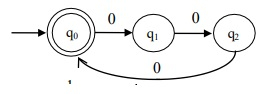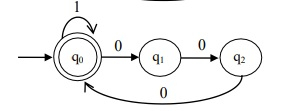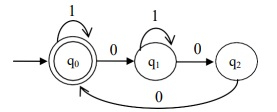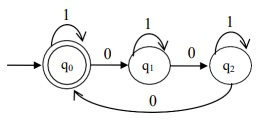# Design a DFA accepting a language L having number of zeros in multiples of 3

## Problem

Construct a deterministic finite automata (DFA) that accepts a language L which has the number of zero’s is of multiple of 3 over an alphabet ∑=”{0,1}.

## Solution

If input is: 000
Output is: string is accepted

Because here the number of zero’s is multiple of 3.

### Designing DFA

In order to construct the DFA, follow the below mentioned steps −

Step 1 -Valid inputs: 000, 000000, 09 , 012 , …

Step 2 -Valid inputs: 1, 1000, 100000, …

Step 3 -Valid inputs: 10100, 11000, 101100, …

Step 4 -101010, 1101010, 1101110110, …

Invalid inputs − 0,00,10000,01011, …